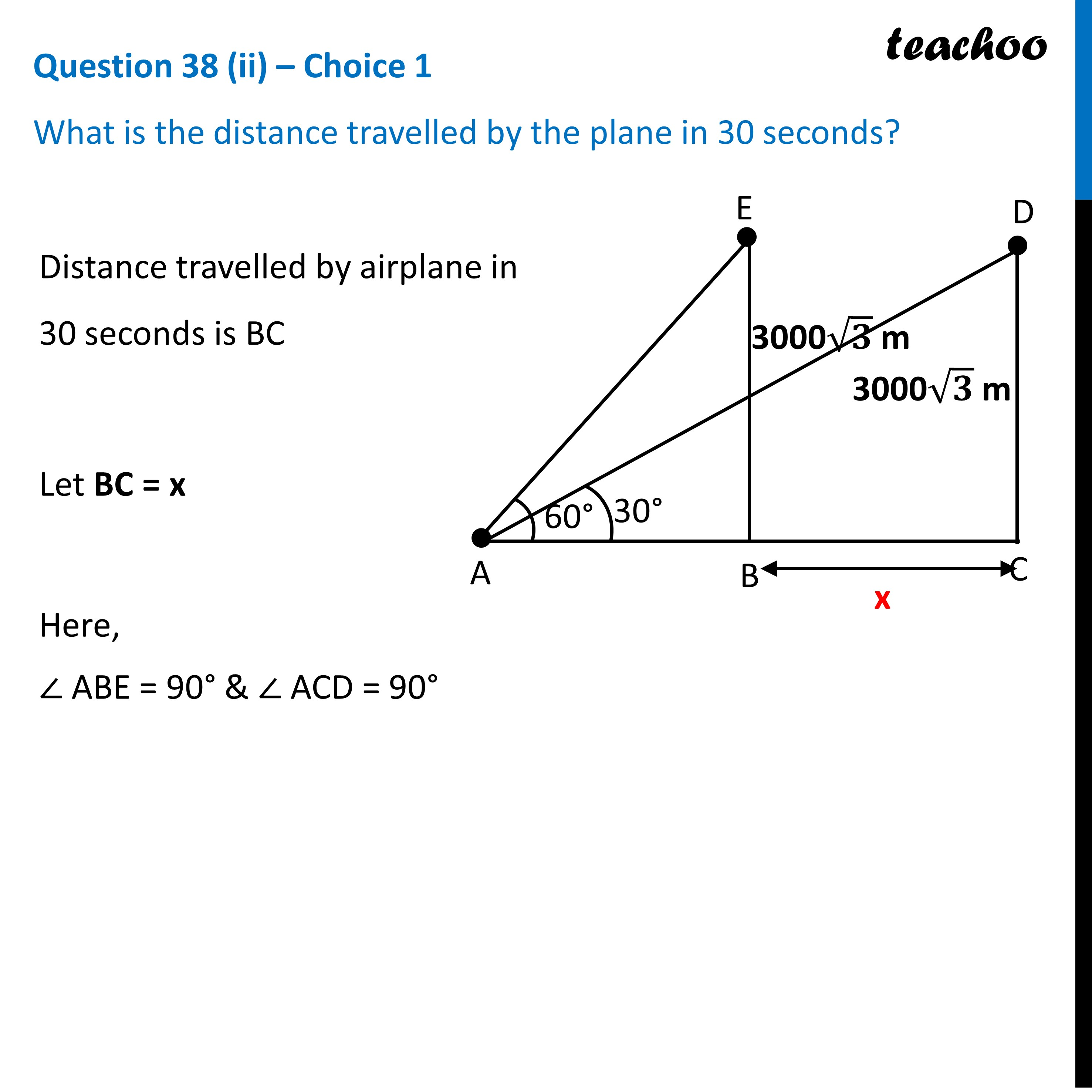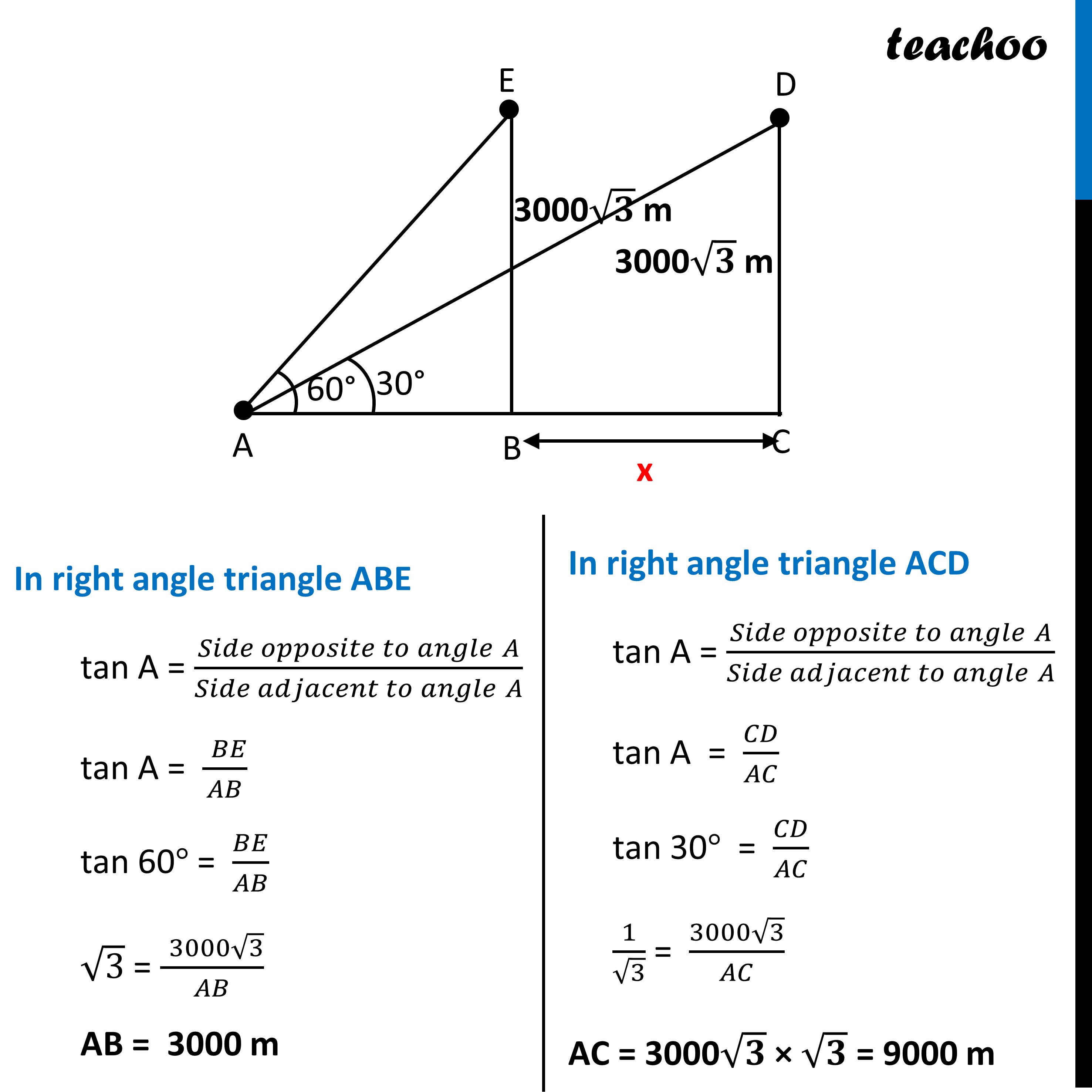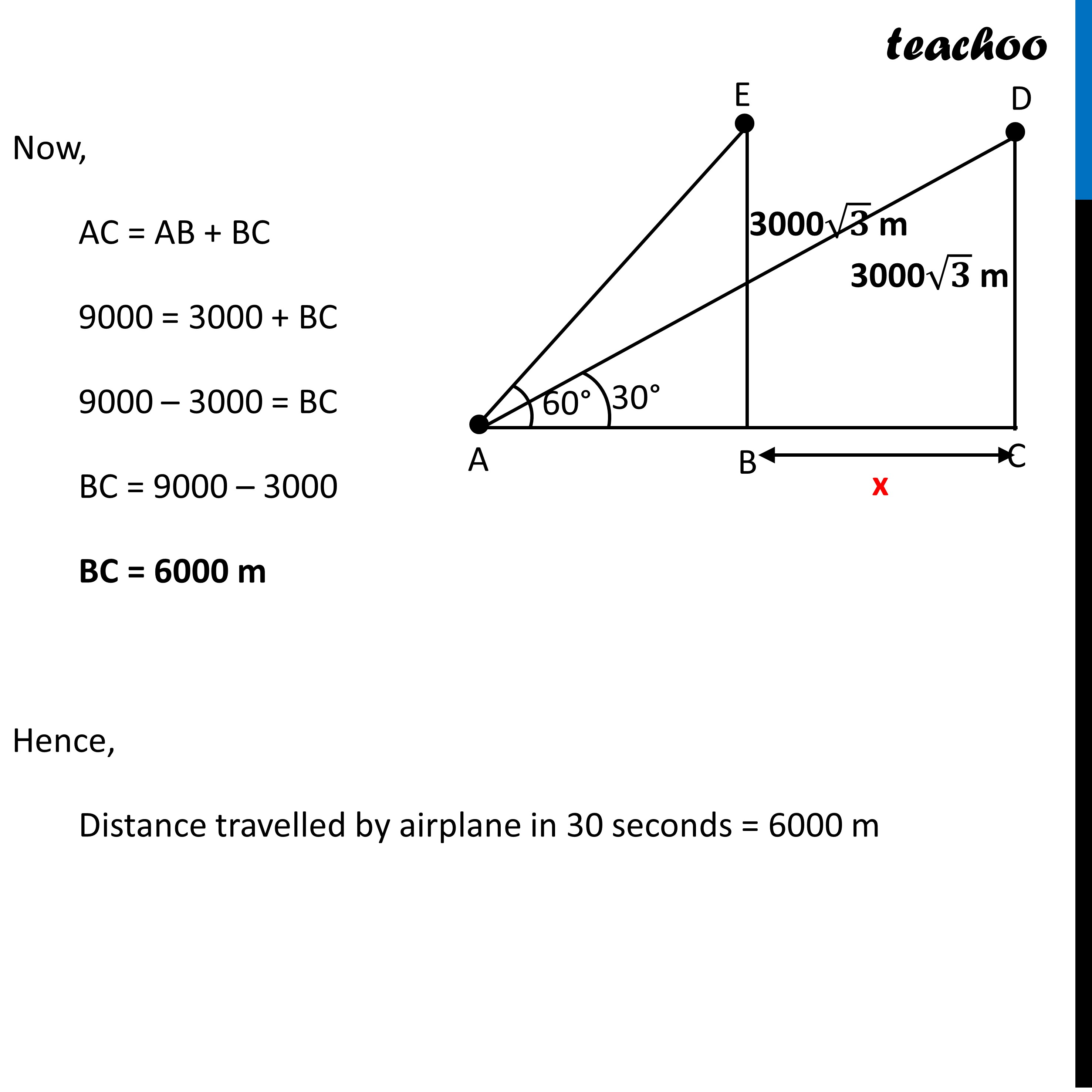CBSE Class 10 Sample Paper for 2023 Boards - Maths Standard

Class 10
Solutions of Sample Papers for Class 10 Boards

## What is the distance travelled by the plane in 30 seconds?Get live Maths 1-on-1 Classs - Class 6 to 12

### Transcript

Question 38 (ii) – Choice 1 What is the distance travelled by the plane in 30 seconds? Distance travelled by airplane in 30 seconds is BC Let BC = x Here, ∠ ABE = 90° & ∠ ACD = 90° In right angle triangle ABE tan A = (𝑆𝑖𝑑𝑒 𝑜𝑝𝑝𝑜𝑠𝑖𝑡𝑒 𝑡𝑜 𝑎𝑛𝑔𝑙𝑒" " 𝐴)/(𝑆𝑖𝑑𝑒 𝑎𝑑𝑗𝑎𝑐𝑒𝑛𝑡 𝑡𝑜 𝑎𝑛𝑔𝑙𝑒" " 𝐴) tan A = (" " 𝐵𝐸)/𝐴𝐵 tan 60° = 𝐵𝐸/𝐴𝐵 √3 = (" " 3000√3)/𝐴𝐵 AB = 3000 m In right angle triangle ACD tan A = (𝑆𝑖𝑑𝑒 𝑜𝑝𝑝𝑜𝑠𝑖𝑡𝑒 𝑡𝑜 𝑎𝑛𝑔𝑙𝑒" " 𝐴)/(𝑆𝑖𝑑𝑒 𝑎𝑑𝑗𝑎𝑐𝑒𝑛𝑡 𝑡𝑜 𝑎𝑛𝑔𝑙𝑒" " 𝐴) tan A = 𝐶𝐷/𝐴𝐶 tan 30° = 𝐶𝐷/𝐴𝐶 1/√3 = (3000√3)/𝐴𝐶 AC = 3000√𝟑 × √𝟑 = 9000 m Now, AC = AB + BC 9000 = 3000 + BC 9000 – 3000 = BC BC = 9000 – 3000 BC = 6000 m Hence, Distance travelled by airplane in 30 seconds = 6000 m